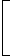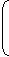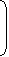The Eduladder is a community of students, teachers, and programmers just interested to make you pass any exams. So we help you to solve your academic and programming questions fast.
Watch related videos of your favorite subject.
Connect with students from different parts of the world.
See Our team
Wondering how we keep quality?
Got unsolved questions?

You are here:Open notes-->Interview-->Aptitude-Test--Problems-on-Trains--3

# How to study this subject

21.

How many seconds will a 500 metre long train take to cross a man walking with a speed of 3 km/hr in the direction of the moving train if the speed of the train is 63 km/hr?

 A. 25 B. 30 C. 40 D. 45

Explanation:

Speed of the train relative to man= (63 - 3) km/hr
= 60 km/hr
 =60 x 5m/sec 18
 =50m/sec. 3Time taken to pass the man
 =500 x 3sec 50
= 30 sec.

22.

Two goods train each 500 m long, are running in opposite directions on parallel tracks. Their speeds are 45 km/hr and 30 km/hr respectively. Find the time taken by the slower train to pass the driver of the faster one.

 A. 12 sec B. 24 sec C. 48 sec D. 60 sec

Explanation:

Relative speed == (45 + 30) km/hr
 =75 x 5m/sec 18
 =125m/sec. 6

We have to find the time taken by the slower train to pass the DRIVER of the faster train and not the complete train.

So, distance covered = Length of the slower train.

Therefore, Distance covered = 500 m.Required time =500 x 6= 24 sec. 125

23.

Two trains are running in opposite directions with the same speed. If the length of each train is 120 metres and they cross each other in 12 seconds, then the speed of each train (in km/hr) is:

 A. 10 B. 18 C. 36 D. 72

Explanation:

Let the speed of each train be x m/sec.

Then, relative speed of the two trains = 2x m/sec.

 So, 2x = (120 + 120) 122x = 20x = 10.Speed of each train = 10 m/sec =10 x 18km/hr = 36 km/hr. 5

24.

Two trains of equal lengths take 10 seconds and 15 seconds respectively to cross a telegraph post. If the length of each train be 120 metres, in what time (in seconds) will they cross each other travelling in opposite direction?

 A. 10 B. 12 C. 15 D. 20

Explanation:

 Speed of the first train =120m/sec = 12 m/sec. 10
 Speed of the second train =120m/sec = 8 m/sec. 15

Relative speed = (12 + 8) = 20 m/sec.Required time =(120 + 120)sec = 12 sec. 20

25.

A train 108 m long moving at a speed of 50 km/hr crosses a train 112 m long coming from opposite direction in 6 seconds. The speed of the second train is:

 A. 48 km/hr B. 54 km/hr C. 66 km/hr D. 82 km/hr

Explanation:

Let the speed of the second train be x km/hr.

Relative speed= (x + 50) km/hr
 =(x + 50) x 5m/sec 18
 =250 + 5xm/sec. 18

Distance covered = (108 + 112) = 220 m.220= 6250 + 5x18250 + 5x = 660x = 82 km/hr.

26.

Two trains are running at 40 km/hr and 20 km/hr respectively in the same direction. Fast train completely passes a man sitting in the slower train in 5 seconds. What is the length of the fast train?

A.23 mB.
 23 2 m 9
C.
 27 7 m 9
D.29 m

Explanation:

 Relative speed = (40 - 20) km/hr =20 x 5m/sec =50m/sec. 18 9Length of faster train =50 x 5m = 250 m = 27 7 m. 9 9 9

27.

A train overtakes two persons who are walking in the same direction in which the train is going, at the rate of 2 kmph and 4 kmph and passes them completely in 9 and 10 seconds respectively. The length of the train is:

 A. 45 m B. 50 m C. 54 m D. 72 m

Explanation:

 2 kmph =2 x 5m/sec = 5 m/sec. 18 9
 4 kmph =4 x 5m/sec = 10 m/sec. 18 9

Let the length of the train be x metres and its speed by y m/sec.

Then,x= 9 andx= 10.
 y - 5 9
 y - 10 99y - 5 = x and 10(9y - 10) = 9x9y - x = 5 and 90y - 9x = 100.

On solving, we get: x = 50.Length of the train is 50 m.

28.

A train overtakes two persons walking along a railway track. The first one walks at 4.5 km/hr. The other one walks at 5.4 km/hr. The train needs 8.4 and 8.5 seconds respectively to overtake them. What is the speed of the train if both the persons are walking in the same direction as the train?

 A. 66 km/hr B. 72 km/hr C. 78 km/hr D. 81 km/hr

Explanation:

 4.5 km/hr =4.5 x 5m/sec = 5 m/sec = 1.25 m/sec, and 18 4
 5.4 km/hr =5.4 x 5m/sec = 3 m/sec = 1.5 m/sec. 18 2

Let the speed of the train be x m/sec.

Then, (x - 1.25) x 8.4 = (x - 1.5) x 8.58.4x - 10.5 = 8.5x - 12.750.1x = 2.25x = 22.5Speed of the train =22.5 x 18km/hr = 81 km/hr. 5

29.

A train travelling at 48 kmph completely crosses another train having half its length and travelling in opposite direction at 42 kmph, in 12 seconds. It also passes a railway platform in 45 seconds. The length of the platform is

 A. 400 m B. 450 m C. 560 m D. 600 m

Explanation:

Let the length of the first train be x metres.

 Then, the length of the second train isxmetres. 2
 Relative speed = (48 + 42) kmph =90 x 5m/sec = 25 m/sec. 18[x + (x/2)] = 12 or 3x = 300     or     x = 200. 25 2Length of first train = 200 m.

Let the length of platform be y metres.

 Speed of the first train =48 x 5m/sec = 40 m/sec. 18 3(200 + y) x 3 = 45 40600 + 3y = 1800y = 400 m.

30.

Two stations A and B are 110 km apart on a straight line. One train starts from A at 7 a.m. and travels towards B at 20 kmph. Another train starts from B at 8 a.m. and travels towards A at a speed of 25 kmph. At what time will they meet?

 A. 9 a.m. B. 10 a.m. C. 10.30 a.m. D. 11 a.m.

Explanation:

Suppose they meet x hours after 7 a.m.

Distance covered by A in x hours = 20x km.

Distance covered by B in (x - 1) hours = 25(x - 1) km.20x + 25(x - 1) = 11045x = 135x = 3.

So, they meet at 10 a.m.

31.

Two, trains, one from Howrah to Patna and the other from Patna to Howrah, start simultaneously. After they meet, the trains reach their destinations after 9 hours and 16 hours respectively. The ratio of their speeds is:

 A. 2 : 3 B. 4 : 3 C. 6 : 7 D. 9 : 16

# Previous year question papers

#### Editors

arunwebberarunwebberarunwebber

## Tool box

Edit this note | Upvote | Down vote | Questions

### Watch more videos from this user Here

Learn how to upload a video over here